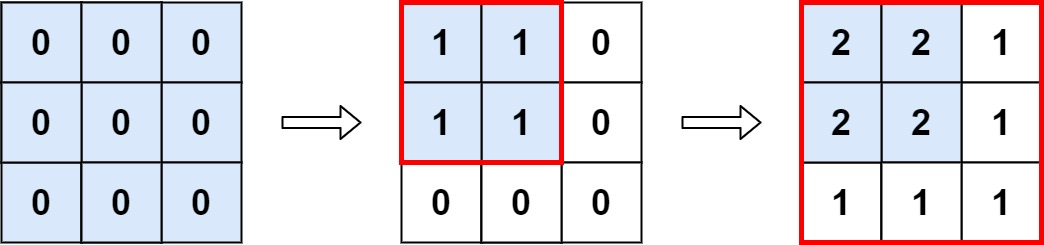Easy
19
40.1% Acceptance

In this lab, you will be working on a problem related to matrices and operations on them. Your task is to implement the `maxCount` function that takes an `m x n` matrix `M` initialized with all `0`'s and an array of operations `ops`, where `ops[i] = [ai, bi]`. It means `M[x][y]` should be incremented by one for all `0 <= x < ai` and `0 <= y < bi`. The goal is to count and return the number of maximum integers in the matrix after performing all the operations.

Example 1:``maxCount(3, 3, [[2, 2], [3, 3]])``

The above function call should return `4`. The maximum integer in `M` is `2`, and there are four of it in the matrix. So return `4`.

Example 2:

``maxCount(3, 3, [[2, 2], [3, 3], [3, 3], [3, 3], [2, 2], [3, 3], [3, 3], [3, 3], [2, 2], [3, 3], [3, 3], [3, 3]]);``

The above function call should return `4`.

Example 3:

If there are no operations in the array, the function should return `m * n`.

``maxCount(3, 3, [])``

The above function call should return `9`.

Constraints:

• `1 <= m, n <= 4 * 104`
• `0 <= ops.length <= 104`
• `ops[i].length == 2`
• `1 <= ai <= m`
• `1 <= bi <= n`

## Challenges

Finish the implementation of the `maxCount` function and use ESM import/export everywhere. Make sure to export the `maxCount` function, which will be used in the evaluation script.

1. Implement the `maxCount` function, which accepts three arguments - `m`, `n`, and `ops`.

Make sure you default export the function